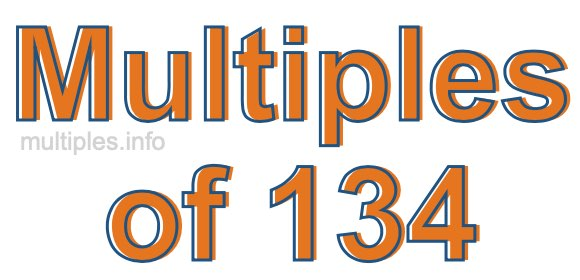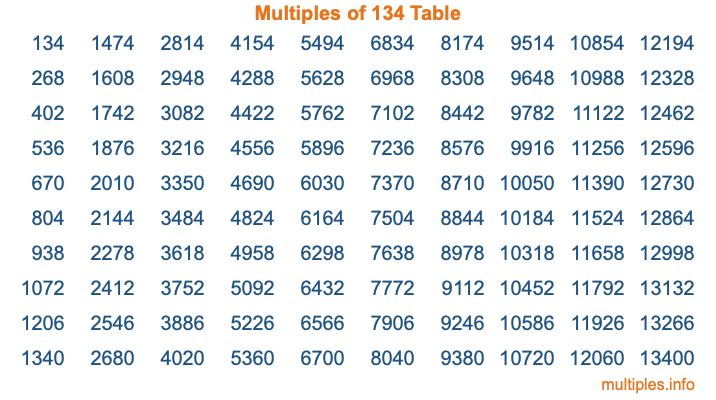Multiples of 134Welcome to the Multiples of 134 page. Here we will first teach you everything you will ever need to know about the multiples of 134, and then give you a study guide summary of everything we taught you to make sure you remember it all. Use this page to look up facts and learn information about the multiples of 134. This page will make you a multiples of one hundred thirty-four expert!

Definition of Multiples of 134
Multiples of 134 are all the numbers that when divided by 134 equal an integer. Each of the multiples of 134 are called a multiple. A multiple of 134 is created by multiplying 134 by an integer.

Therefore, to create a list of multiples of 134, you start with 1 multiplied by 134, then 2 multiplied by 134, then 3 multiplied by 134, and so on for as long as you want. Thus, the list of the first five multiples of 134 is 134, 268, 402, 536, and 670. To see a larger list of multiples of 134, see the printable image of Multiples of 134 further down on this page. We also have a category where you can choose any nth multiple of 134.

Multiples of 134 Checker
The Multiples of 134 Checker below checks to see if any number of your choice is a multiple of 134. In other words, it checks to see if there is any number (integer) that when multiplied by 134 will equal your number. To do that, we divide your number by 134. If the the quotient is an integer, then your number is a multiple of 134.

Is  a multiple of 134?

Least Common Multiple of 134 and ...
A Least Common Multiple (LCM) is the lowest multiple that two or more numbers have in common. This is also called the smallest common multiple or lowest common multiple and is useful to know when you are adding our subtracting fractions. Enter one or more numbers below (134 is already entered) to find the LCM.

Check out our LCM Calculator if you need more details about the Least Common Multiple or if you need the LCM for different numbers for adding and subtraction fractions.

nth Multiple of 134
As we stated above, 134 is the first multiple of 134, 268 is the second multiple of 134, 402 is the third multiple of 134, and so on. Enter a number below to find the nth multiple of 134.

th multiple of 134

Multiples of 134 vs Factors of 134
134 is a multiple of 134 and a factor of 134, but that is where the similarities end. All postive multiples of 134 are 134 or greater than 134. All positive factors of 134 are 134 or less than 134.

Below is the beginning list of multiples of 134 and the factors of 134 so you can compare:

Multiples of 134: 134, 268, 402, 536, 670, etc.

Factors of 134: 1, 2, 67, 134

As you can see, the multiples of 134 are all the numbers that you can divide by 134 to get a whole number. The factors of 134, on the other hand, are all the whole numbers that you can multiply by another whole number to get 134.

It's also interesting to note that if a number (x) is a factor of 134, then 134 will also be a multiple of that number (x).

Multiples of 134 vs Divisors of 134
The divisors of 134 are all the integers that 134 can be divided by evenly. Below is a list of the divisors of 134.

Divisors of 134: 1, 2, 67, 134

The interesting thing to note here is that if you take any multiple of 134 and divide it by a divisor of 134, you will see that the quotient is an integer.

Multiples of 134 Table
Below is an image of the first 100 multiples of 134 in a table. The table is in chronological order, column by column. The first column has the first ten multiples of 134, the second column has the next ten multiples of 134, and so on.The Multiples of 134 Table is also referred to as the 134 Times Table or Times Table of 134. You are welcome to print out our table for your studies.

Negative Multiples of 134
Although not often discussed or needed in math, it is worth mentioning that you can make a list of negative multiples of 134 by multiplying 134 by -1, then by -2, then by -3, and so on, to get the following list of negative multiples of 134:

-134, -268, -402, -536, -670, etc.

Multiples of 134 Summary
Below is a summary of important Multiples of 134 facts that we have discussed on this page. To retain the knowledge on this page, we recommend that you read through the summary and explain to yourself or a study partner why they hold true.

There are an infinite number of multiples of 134.

A multiple of 134 divided by 134 will equal a whole number.

134 divided by a factor of 134 equals a divisor of 134.

The nth multiple of 134 is n times 134.

The largest factor of 134 is equal to the first positive multiple of 134.

134 is a multiple of every factor of 134.

134 is a multiple of 134.

A multiple of 134 divided by a divisor of 134 equals an integer.

134 divided by a divisor of 134 equals a factor of 134.

Any integer times 134 will equal a multiple of 134.

Multiples of a Number
Here you can get the multiples of another number, all with the same attention to detail as we did for multiples of 134 on this page.

Multiples of
Multiples of 135
Did you find our page about multiples of one hundred thirty-four educational? Do you want more knowledge? Check out the multiples of the next number on our list!